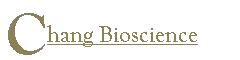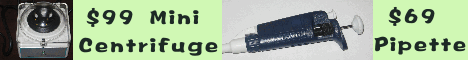Help: PCR Primer Design

 1. Why can't I paste in my sequences? 2. What input sequence formats does Primo accept? 3. My target is a human gene, do I need the stand-alone version? 4. For calculating the melting temperature Tm, should I always use the Nearest Neighbor formula? 5. How do I calculate Tm for a known primer sequence using the Nearest Neighbor formula? 6. Why there are only two formulae for calculating Tm? 7. How big are the differences for the melting temperature calculated using the two formulae? 8. How do I use the batch mode? 9. How do I calculate the melting temperature for degenerate primers? 10. How do I design the multiplex PCR primers? 11. The program keeps on "searching", why? 12. I used Primo Degenerate, and don't get any results, why? 13. I can not find the 5'-position for Primo Degenerate 14. Why can't I find any multiplex primer sets using Primo Multiplex? 15. What is the primer concentration Primo uses for calculating Tm? 16. What is the primer concentration Primo Degenerate uses for calculating Tm? 17. What is the annealing temperature one should use for a PCR reaction? 18. What is the annealing temperature one should use for a degenerate PCR reaction? 19. What is the difference between Primo Degenerate and Diverse? 20. When should I use the "avoid background primering" option? 21. Does the "avoid background primering" work? 22. I tried to paste in a large chunk of data, nothing happens. Why? 23. How can I check whether my set of primers form primer-primer dimers?
##### 1. Why can't I paste in my sequences?
To copy and paste your sequences into the input windows of Primo, you may need to use Control(Ctrl)+c or Apple+c for copy, and Control(Ctrl)+v or Apple+v for paste. To copy, highlight the sequences and press simultaneously the Control(Ctrl) and the c keys. To paste, first mouse click in the input window, then press simultaneously the Control(Ctrl) and the v keys.

Mac OSX 10 IE5.1 has a bug, it does not allow copy/paste into a Java text field, thus you will not be able to import a new sequence. Attempting copy/paste may kill your browser. Later Mac IE versions should work fine. Stand-alone version of Primo does not use IE, so copy/paste works fine.

##### 2. What input sequence formats does Primo accept?

Primo accepts sequences in the single-letter codes. Degenerate codes are OK. It will discard any numbers, white spaces, and any letters that are not recognized as codes for nucleotide sequences.

##### 3. My target is a human gene, do I need the stand-alone version?
You don't need the stand-alone version if you don't want to use the "Avoid background priming" option. If your target is a human gene, you can use the free Primo online. Please check the User Instructions for details.
##### 4. For calculating the melting temperature Tm, should I always use the Nearest Neighbor formula?
The nearest neighbor formula is more accurate for calculating the primer melting temperature. But it is not clear whether it is a better base for calculating the PCR annealing temperature.
##### 5. How do I calculate Tm for a known primer sequence using the Nearest Neighbor formula?
Type or paste in your primer sequence in the "2nd primer" field, select the Tm formula, then click in the "2nd primer" field.

##### 6. Why there are only two formulae for calculating Tm?
We present the easiest and the most accurate. Others may be viewed as approximations of the Nearest Neighbor model which was built on a large set of data.
##### 7. How big are the differences for the melting temperature calculated using the two formulae?
Click here to see the melting temperatures for some commonly used PCR and sequencing primers.
##### 8. How do I use the batch mode?
See user instruction for details.
##### 9. How do I calculate the melting temperature for degenerate primers?
Degenerate primers have lower effective primer concentration. If you select the Nearest Neighbor formula, this correction due to degeneracy has been taken into account. Otherwise, the rule-of-thumb is that for each 2 fold degeneracy, the melting temperature should decrease 1 degree, i.e., the melting temperature for a 1000-fold degenerate primer should be ~10 degree lower than calculated without the correction. Click here for more info.
##### 10. How do I design the multiplex PCR primers?
See user instruction for details.
##### 11. The program keeps on "searching", why?
Check whether you have selected the proper options for your input sequence.
##### 12. I used Primo Degenerate, and don't get any results, why?
Depending on your sequences, the degeneracy may be so high that the melting temperature is low. Lower the melting temperature to get a primer prediction then optimize other parameters to increase stringency (melting temperature).
##### 13. I can not find the 5'-position for Primo Degenerate in the input sequence.
The reference is the alignment: 60 letters per row. Don't confuse with the numbering for a particular gene.
##### 14. Why can't I find any multiplex primer sets using Primo Multiplex?
Most frequently the reason is that the regions you selected for forward and reverse primers don't have matching %GC or the %GC for these regions don't match the value you selected. Change %GC or expand the regions.
##### 15. What is the primer concentration Primo uses for calculating Tm?
The primer concentration is 200 nM.
##### 16. What is the primer concentration Primo Degenerate uses for calculating Tm?
The primer concentration is 200 nM, although the primer concentration used in degenerate PCR could be as high as 2 mM.
##### 17. What is the annealing temperature one should use for a PCR reaction?
Primo calculates the melting temperature. Usually the annealing temperature for a PCR reaction is 2-6 °C lower than the melting temperature.
##### 18. What is the annealing temperature one should use for a degenerate PCR reaction?
If using degenerate primers and the AT=2 CG=4 °C formula for calculating the melting temperature, the annealing temperature is frequently 4-10 °C lower even though the primer concentration may be 10 times higher. Tm calculated using the Nearest Neighbor model is lower because of the degeneracy. Suggested annealing temperature is 2 °C higher to 4 °C lower than the melting temperature for the normal (200 nM) primer concentration. If higher primer concentration is used, melting temperature increases ~1 °C for each doubling of the primer concentration.
##### 19. What is the difference between Primo Degenerate and Diverse?
Both can be used to design a pair of primers to amplify a family of genes. Primers are selected from the conserved regions, i.e., least degenerate regions. Diverse presents the most diverse (degenerate) products first and ignored products that are conserved in all family members.
##### 20. When should I use the "avoid background primering" option?
The option is most helpful when the sample is a mixture of DNA (e.g., genomic DNA) or cDNA (e.g., reverse transcription of total RNA). Make sure to select the correct species, because the repeat patterns are different in different species.
##### 21. Does the "avoid background primering" work?
You may try it with the following Alu sequence:
```
1 ggccgggcgc ggtggctcac gcctgtaatc ccagcacttt gggaggccga ggcgggtgga
61 tcacctgagg tcaggagttc gagaccagcc tggccaacat ggtgaaaccc cgtctctact
121 aaaaatacaa aaattagccg ggcgtggtgg cgggcgcctg taatcccagc tactcgggag
181 gctgaggcag gagaatcgct tgaacccggg aggcggaggt tgcagtgagc cgagatcgcg
241 ccactgcact ccagcctggg caacaagagc gaaactccgt ctcaaaaaaa a
```
Check and uncheck the "avoid background primering" option to see the differences. If your sequence contains Alu-like or many other repeat sequences, Primo will not select them as primers.
##### 22. I tried to paste in a large chunk of data, nothing happens. Why?
Your browser has a limit on clip board size. You will need the stand-alone version, which will load large data set from a file.
##### 23. How can I check whether my set of primers form primer-primer dimers?
Click here to start Primo Multiplex. Click on the "Check" button next to "Check primer-primer dimers." A new window will appear. Input your primer sequences and click on "Go" in the new window.

Home Products Order Contact